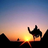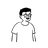V2EX = way to explore
V2EX 是一个关于分享和探索的地方

Learn Python the Hard Way
Python Sites
PyPI - Python Package Index
http://diveintopython.org/toc/index.html
Pocoo

PyPy
Celery
Jinja2
gevent
pyenv
virtualenv
Stackless Python
Beautiful Soup

Green Unicorn
Sentry
Shovel
Pyflakes
pytest
Python 编程
pep8 Checker
Styles
PEP 8
Code Style from The Hitchhiker's Guide
V2EX  ›  Python

# 求教一条 Python 中使用的 sql 语句

youthfire · 123 天前 · 1973 次点击

Python 业余编程爱好者，查了好久都没解决，来请求下帮助

condi1 = 'code like' + '"%' + str(code) + '%"'
condi2 = 'code like' + '"%' + str(code2) + '%"'
df = pd.DataFrame(pd.read_sql('select * from f63 where ' + condi1 + ' OR ' + condi2, conn))

condi1 = 'code =' + '"%' + str(code) + '%"'
condi2 = 'code =' + '"%' + str(code2) + '%"'
df = pd.DataFrame(pd.read_sql('select * from f63 where ' + condi1 + ' OR ' + condi2, conn))

df = pd.DataFrame(pd.read_sql("select * from f63 where code = ? OR code = ? AND engine like ?", conn, params=[str(code), str(code2), '%' + str(keystring2) + '%']))
5 条回复    2022-01-19 10:15:42 +08:001 ila      123 天前 via Android1 用 f format,f"{condi1}"。先 print 出来看组装后的字符串2 youthfire   OP   123 天前 自己发现了两处问题1.改用 code = 时，后面%就不需要了，因为只有在 like 时有效2.安全角度和便捷角度，应该使用参数写法但换了种表达方式，似乎也不见效df = pd.DataFrame(pd.read_sql("select * from f63 where code = ? OR code = ? AND engine like '%?%'", (str(code), str(code2), str(keystring2))), conn)3 youthfire   OP   123 天前 解决了df = pd.DataFrame(pd.read_sql("select * from f63 where code = ? OR code = ? AND engine like ?", conn, params=[str(code), str(code2), '%' + str(keystring2) + '%']))4 dayeye2006199      122 天前1 虽然这边是用在 pandas 里面，但是拼装 sql 语句不是好习惯。5 RRRoger      122 天前1 用 py3 的 f"....." 或者 "...".format()都比你这个+清晰